# IB DP Physics: SL复习笔记3.1.6 Phase Change

### Phase Change

• phase change happens whenever matter changes its state
• During a phase change, thermal energy is transferred to or from a substance
• This energy transfer does not change the temperature of the substance undergoing the phase change
• This means:
• The thermal energy provided (or removed) does not affect the kinetic energy of the molecules within the substance
• Only the potential energy (i.e. the spacing between the atoms or molecules) is affected
• The four main phase changes are:
• Melting - i.e. when a substance changes from solid to liquid as it absorbs thermal energy
• Freezing - i.e. when a substance changes from liquid to solid as it releases thermal energy
• Vaporisation (or boiling) - i.e. when a substance changes from liquid to gas as it absorbs thermal energy
• Condensation - i.e. when a substance changes from gas to liquid as it releases thermal energy

#### Water

• Each substance has its own melting (or freezing) and boiling points
• For example, the freezing point of water is 0°C and its boiling point is 100°C
• Possible phase changes of water include:
• Solid ice melting into liquid water at 0°C
• Liquid water boiling and changing into gaseous water vapour at 100°C
• Both these changes happen when thermal energy is absorbed
• If thermal energy is released from water vapour at 100°C, it condenses back into water
• If water continues to release thermal energy, it cools down until it reaches 0°C and freezes into ice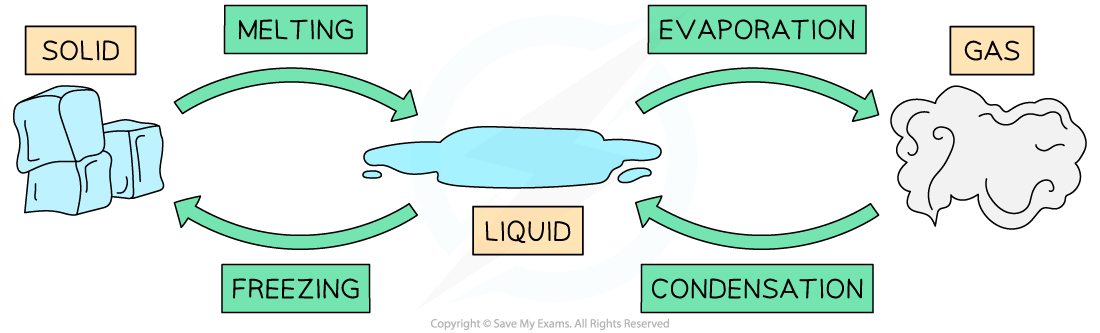Phase changes for water

• Melting and freezing happen at the melting freezing point of a substance
• Vaporisation and condensation happen at the boiling point of a substance

### Phase Change Graphs

• heating or cooling curve shows how the temperature of a substance changes with time
• The 'flat' sections of the graph indicate that there is no change in temperature over time, hence the substance is undergoing a phase change
• The thermal energy supplied to or removed from the substance only affects the potential energy of the particles
• The regions of the graph that are not flat indicate that the substance is being heated or cooled down
• The thermal energy supplied to or removed from the substance changes the average kinetic energy of the particles, hence resulting in an overall change in temperature of the substance

#### Heating Curves

• As energy is being supplied to a solid substance, its temperature increases until it reaches its melting point
• The temperature remains constant until the substance has melted completely
• If energy continues to be supplied, the liquid substance warms up until the boiling point is reached, and the substance vaporises
• Then, the temperature of the gas increases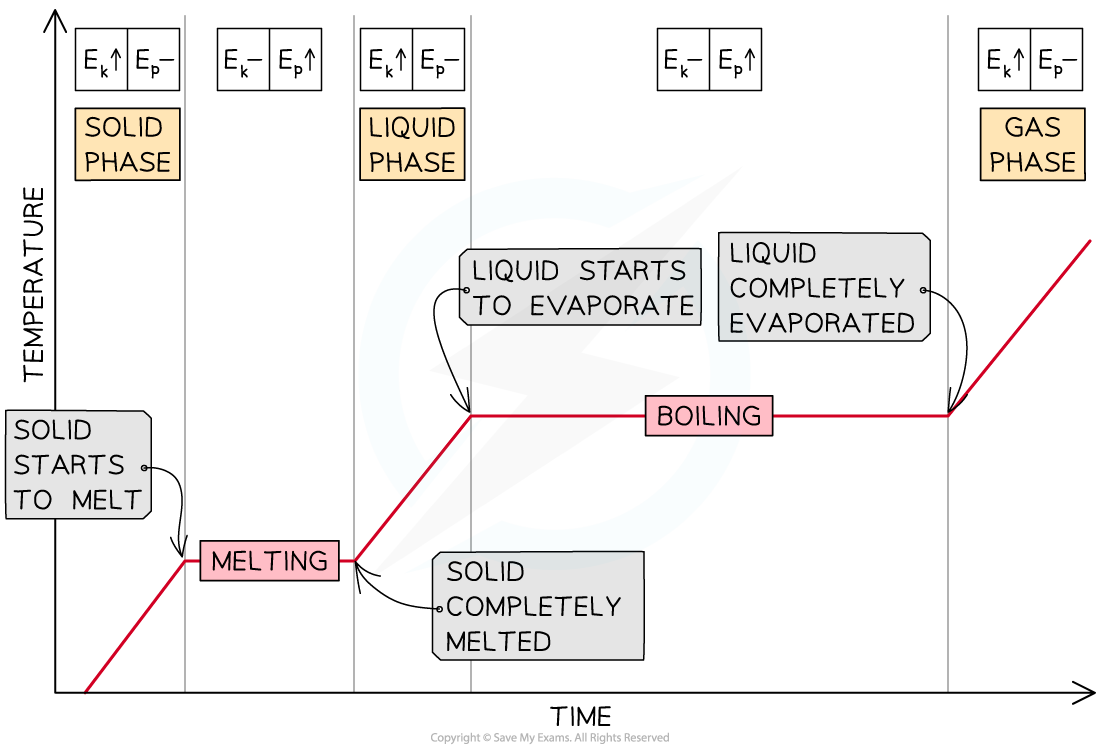#### Cooling Curves

• As energy is being removed from a gaseous substance, its temperature decreases until the boiling point is reached
• The temperature remains constant until the substance has condensed completely
• If energy continues to be removed, the liquid substance cools down until its freezing point and changes into a solid
• Then, the temperature of the solid decreases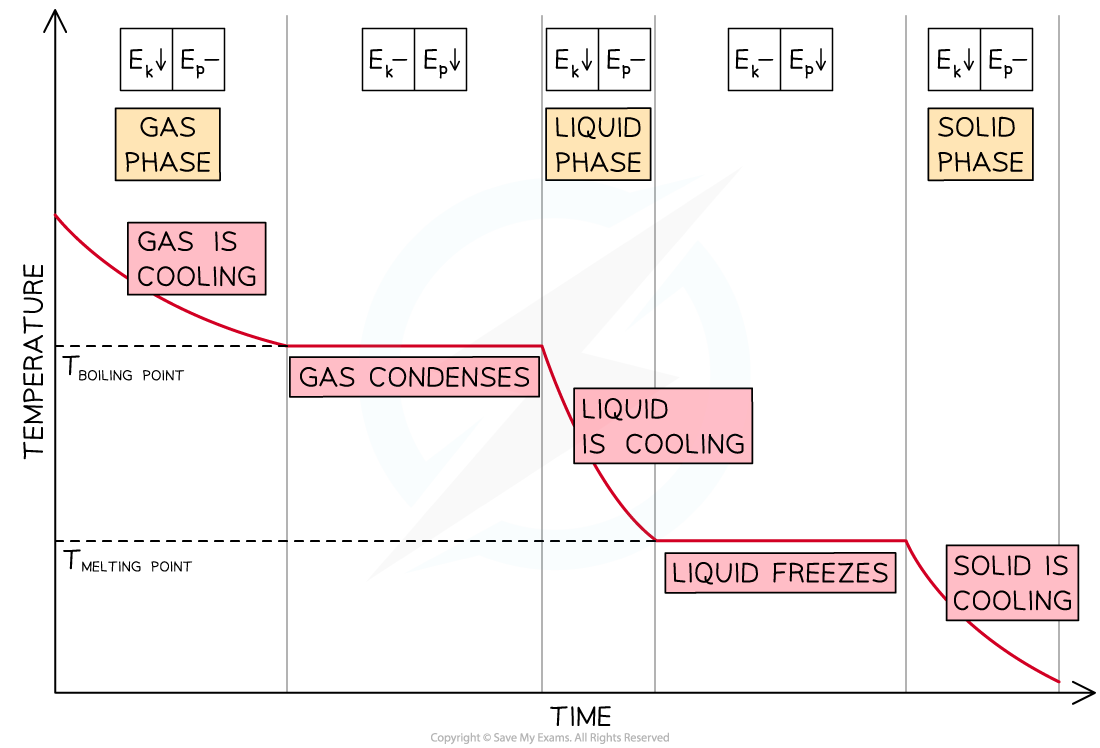• Heating or cooling curves can also display how the temperature of a substance changes with energy
• In the following worked example, energy (in J) is plotted on the x-axis instead of time

#### Worked Example

The graph below is the heating curve for a 25 g cube of ice being heated at a constant rate. Calculate:

• The specific heat capacity of water in its liquid phase
• The specific latent heat of vaporisation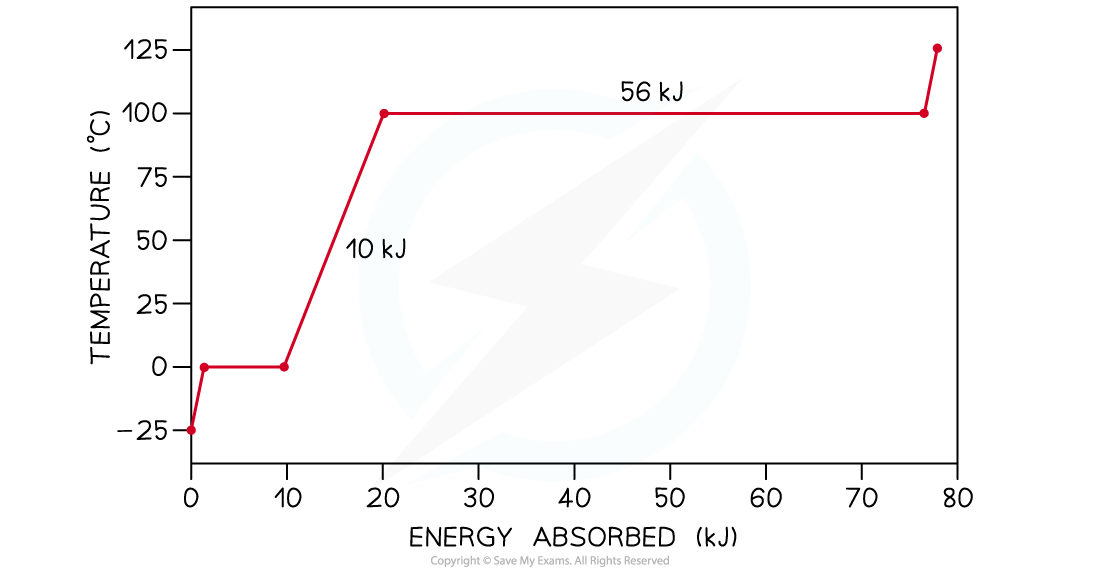Step 1: Write down the mass m of ice in kilograms (kg)

m = 25 g = 0.025 kg

Step 2:  Read from the graph the amount of energy E1 being supplied to the water in its liquid phase as it warms up

• The first flat section of the graph indicates the change of phase of ice into water
• The non-flat region that follows is the one relating to water in its liquid phase being heated up

E1 = 20 kJ – 10 kJ = 10 kJ

Step 3: Convert this energy from kilojoules into joules

E1 = 10 kJ = 10000 J

Step 4:  Read from the graph the change in temperature ΔT1 of the water as it warms up

ΔT1 = 100°C – 0°C = 100°C

Step 5: Write down the equation linking thermal energy E1 to mass m, specific heat capacity c and change in temperature ΔT1

E1 = mcΔT1

Step 6: Solve for the specific heat capacity c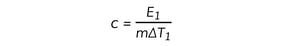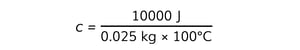c = 4000 J kg–1 °C–1

Step 7: Read from the graph the amount of energy E2 being supplied to the water as it changes into a gas at 100°C

• The second flat section of the graph indicates the change of phase of water into water vapour
• This energy must be converted from kilojoules (kJ) into joules (J)

E2= 56 kJ = 56000 J

Step 8: Write down the equation linking thermal energy E2 to mass m and specific latent heat of vaporisation Lv

E2 = mLv

Step 9: Solve for the specific latent heat of fusion Lv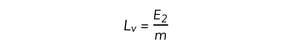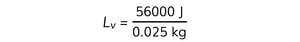L= 2.2 × 106 J kg–1